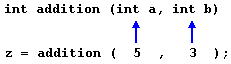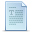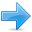# Functions (II)

### Arguments passed by value and by reference.

Until now, in all the functions we have seen, the arguments passed to the functions have been passed by value. This means that when calling a function with parameters, what we have passed to the function were copies of their values but never the variables themselves. For example, suppose that we called our first function addition using the following code:

 ``12`` ``````int x=5, y=3, z; z = addition ( x , y );``````

What we did in this case was to call to function addition passing the values of x and y, i.e. 5 and 3 respectively, but not the variables x and y themselves.This way, when the function addition is called, the value of its local variables a and b become 5 and 3 respectively, but any modification to either a or b within the function addition will not have any effect in the values of x and y outside it, because variables x and y were not themselves passed to the function, but only copies of their values at the moment the function was called.

But there might be some cases where you need to manipulate from inside a function the value of an external variable. For that purpose we can use arguments passed by reference, as in the function duplicate of the following example:

 ``````// passing parameters by reference #include using namespace std; void duplicate (int& a, int& b, int& c) { a*=2; b*=2; c*=2; } int main () { int x=1, y=3, z=7; duplicate (x, y, z); cout << "x=" << x << ", y=" << y << ", z=" << z; return 0; }`````` `x=2, y=6, z=14`

The first thing that should call your attention is that in the declaration of duplicate the type of each parameter was followed by an ampersand sign (&). This ampersand is what specifies that their corresponding arguments are to be passed by reference instead of by value.

When a variable is passed by reference we are not passing a copy of its value, but we are somehow passing the variable itself to the function and any modification that we do to the local variables will have an effect in their counterpart variables passed as arguments in the call to the function.To explain it in another way, we associate a, b and c with the arguments passed on the function call (x, y and z) and any change that we do on a within the function will affect the value of x outside it. Any change that we do on b will affect y, and the same with c and z.

That is why our program's output, that shows the values stored in x, y and z after the call to duplicate, shows the values of all the three variables of main doubled.

If when declaring the following function:

 ``1`` ``void duplicate (int& a, int& b, int& c)``

we had declared it this way:

 ``1`` ``void duplicate (int a, int b, int c)``

i.e., without the ampersand signs (&), we would have not passed the variables by reference, but a copy of their values instead, and therefore, the output on screen of our program would have been the values of x, y and z without having been modified.

Passing by reference is also an effective way to allow a function to return more than one value. For example, here is a function that returns the previous and next numbers of the first parameter passed.

 ``````// more than one returning value #include using namespace std; void prevnext (int x, int& prev, int& next) { prev = x-1; next = x+1; } int main () { int x=100, y, z; prevnext (x, y, z); cout << "Previous=" << y << ", Next=" << z; return 0; }`````` `Previous=99, Next=101 `

### Default values in parameters.

When declaring a function we can specify a default value for each of the last parameters. This value will be used if the corresponding argument is left blank when calling to the function. To do that, we simply have to use the assignment operator and a value for the arguments in the function declaration. If a value for that parameter is not passed when the function is called, the default value is used, but if a value is specified this default value is ignored and the passed value is used instead. For example:

 ``````// default values in functions #include using namespace std; int divide (int a, int b=2) { int r; r=a/b; return (r); } int main () { cout << divide (12); cout << endl; cout << divide (20,4); return 0; }`````` ```6 5```

As we can see in the body of the program there are two calls to function divide. In the first one:

 ``1`` ``divide (12)``

we have only specified one argument, but the function divide allows up to two. So the function divide has assumed that the second parameter is 2 since that is what we have specified to happen if this parameter was not passed (notice the function declaration, which finishes with int b=2, not just int b). Therefore the result of this function call is 6 (12/2).

In the second call:

 ``1`` ``divide (20,4)``

there are two parameters, so the default value for b (int b=2) is ignored and b takes the value passed as argument, that is 4, making the result returned equal to 5 (20/4).

In C++ two different functions can have the same name if their parameter types or number are different. That means that you can give the same name to more than one function if they have either a different number of parameters or different types in their parameters. For example:

 ``````// overloaded function #include using namespace std; int operate (int a, int b) { return (a*b); } float operate (float a, float b) { return (a/b); } int main () { int x=5,y=2; float n=5.0,m=2.0; cout << operate (x,y); cout << "\n"; cout << operate (n,m); cout << "\n"; return 0; }`````` ```10 2.5```

In this case we have defined two functions with the same name, operate, but one of them accepts two parameters of type int and the other one accepts them of type float. The compiler knows which one to call in each case by examining the types passed as arguments when the function is called. If it is called with two ints as its arguments it calls to the function that has two int parameters in its prototype and if it is called with two floats it will call to the one which has two float parameters in its prototype.

In the first call to operate the two arguments passed are of type int, therefore, the function with the first prototype is called; This function returns the result of multiplying both parameters. While the second call passes two arguments of type float, so the function with the second prototype is called. This one has a different behavior: it divides one parameter by the other. So the behavior of a call to operate depends on the type of the arguments passed because the function has been overloaded.

Notice that a function cannot be overloaded only by its return type. At least one of its parameters must have a different type.

### inline functions.

The inline specifier indicates the compiler that inline substitution is preferred to the usual function call mechanism for a specific function. This does not change the behavior of a function itself, but is used to suggest to the compiler that the code generated by the function body is inserted at each point the function is called, instead of being inserted only once and perform a regular call to it, which generally involves some additional overhead in running time.

The format for its declaration is:

inline type name ( arguments ... ) { instructions ... }

and the call is just like the call to any other function. You do not have to include the inline keyword when calling the function, only in its declaration.

Most compilers already optimize code to generate inline functions when it is more convenient. This specifier only indicates the compiler that inline is preferred for this function.

### Recursivity.

Recursivity is the property that functions have to be called by themselves. It is useful for many tasks, like sorting or calculate the factorial of numbers. For example, to obtain the factorial of a number (n!) the mathematical formula would be:

```n! = n * (n-1) * (n-2) * (n-3) ... * 1
```

more concretely, 5! (factorial of 5) would be:

```5! = 5 * 4 * 3 * 2 * 1 = 120
```

and a recursive function to calculate this in C++ could be:

 ``````// factorial calculator #include using namespace std; long factorial (long a) { if (a > 1) return (a * factorial (a-1)); else return (1); } int main () { long number; cout << "Please type a number: "; cin >> number; cout << number << "! = " << factorial (number); return 0; }`````` ```Please type a number: 9 9! = 362880 ```

Notice how in function factorial we included a call to itself, but only if the argument passed was greater than 1, since otherwise the function would perform an infinite recursive loop in which once it arrived to 0 it would continue multiplying by all the negative numbers (probably provoking a stack overflow error on runtime).

This function has a limitation because of the data type we used in its design (long) for more simplicity. The results given will not be valid for values much greater than 10! or 15!, depending on the system you compile it.

### Declaring functions.

Until now, we have defined all of the functions before the first appearance of calls to them in the source code. These calls were generally in function main which we have always left at the end of the source code. If you try to repeat some of the examples of functions described so far, but placing the function main before any of the other functions that were called from within it, you will most likely obtain compiling errors. The reason is that to be able to call a function it must have been declared in some earlier point of the code, like we have done in all our examples.

But there is an alternative way to avoid writing the whole code of a function before it can be used in main or in some other function. This can be achieved by declaring just a prototype of the function before it is used, instead of the entire definition. This declaration is shorter than the entire definition, but significant enough for the compiler to determine its return type and the types of its parameters.

Its form is:

type name ( argument_type1, argument_type2, ...);

It is identical to a function definition, except that it does not include the body of the function itself (i.e., the function statements that in normal definitions are enclosed in braces { }) and instead of that we end the prototype declaration with a mandatory semicolon (;).

The parameter enumeration does not need to include the identifiers, but only the type specifiers. The inclusion of a name for each parameter as in the function definition is optional in the prototype declaration. For example, we can declare a function called protofunction with two int parameters with any of the following declarations:

 ``12`` ``````int protofunction (int first, int second); int protofunction (int, int);``````

Anyway, including a name for each variable makes the prototype more legible.

 ``````// declaring functions prototypes #include using namespace std; void odd (int a); void even (int a); int main () { int i; do { cout << "Type a number (0 to exit): "; cin >> i; odd (i); } while (i!=0); return 0; } void odd (int a) { if ((a%2)!=0) cout << "Number is odd.\n"; else even (a); } void even (int a) { if ((a%2)==0) cout << "Number is even.\n"; else odd (a); }`````` ```Type a number (0 to exit): 9 Number is odd. Type a number (0 to exit): 6 Number is even. Type a number (0 to exit): 1030 Number is even. Type a number (0 to exit): 0 Number is even.```

This example is indeed not an example of efficiency. I am sure that at this point you can already make a program with the same result, but using only half of the code lines that have been used in this example. Anyway this example illustrates how prototyping works. Moreover, in this concrete example the prototyping of at least one of the two functions is necessary in order to compile the code without errors.

The first things that we see are the declaration of functions odd and even:

 ``12`` ``````void odd (int a); void even (int a);``````

This allows these functions to be used before they are defined, for example, in main, which now is located where some people find it to be a more logical place for the start of a program: the beginning of the source code.

Anyway, the reason why this program needs at least one of the functions to be declared before it is defined is because in odd there is a call to even and in even there is a call to odd. If none of the two functions had been previously declared, a compilation error would happen, since either odd would not be visible from even (because it has still not been declared), or even would not be visible from odd (for the same reason).

Having the prototype of all functions together in the same place within the source code is found practical by some programmers, and this can be easily achieved by declaring all functions prototypes at the beginning of a program.Previous:Functions (I)IndexNext:Arrays